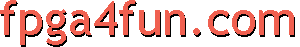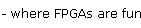## Counters 1 - Binary counters

##### The simplest of counters

A fast and efficient binary counter can be built using a couple of Verilog lines. For example, here's a 32bit counter.

```reg [31:0] cnt;
always @(posedge clk) cnt <= cnt+1;
```

Such counter counts from 0 to 4294967295, and then rolls-back 0 to continue its course. It takes little resources and runs fast in an FPGA thanks to an hidden carry-chain (more on that later). For now, let's see a few variations.

First it's a good idea to explicitly give a starting value, even if it's 0.

```reg [31:0] cnt = 0;
always @(posedge clk) cnt <= cnt+1;
```

Note that if we don't specify a starting value, simulation tools will refuse to work, and some synthesis tools might also change the starting value on their own... so it's a good idea to always specify a starting value. We could also have used an asynchronous reset to specify a starting value, but the simplest way is shown above.

Now if you need more features, here's an example of a 10bit counter (counts up to 1023) that starts counting from 300, and has enable and direction controls.

```reg [9:0] cnt = 10'd300;  // 10bit counter, starts at 300
wire cnt_enable;  // 0 to disable the counter, 1 to enable it
wire cnt_direction;  // 0 to counter backward, 1 to count forward
always @(posedge clk) if(cnt_enable) cnt <= cnt_direction ? cnt+1 : cnt-1;
```

Note that the FPGA synthesis tool has to play some tricks to make the counter start at 300. Flip-flops inside an FPGA always start at 0, so you could think the counter initial value has to be 0... but by putting some well-placed inverters in the logic, any starting value is possible. Inverters are "free" in an FPGA so there is no drawback.

##### Counter tick

Let's say we need a "tick" signal that is asserted once every 1024 clock. Most likely we would create a 10bit counter and some logic to generate the "tick". Let's see how to do that.

First we make our 10bit counter. It counts from 0 to 1023.

```reg [9:0] cnt = 0;
always @(posedge clk) cnt <= cnt+1;
```

Now we could decide that our "tick" is asserted when the counter reaches its maximum value (just before rolling-back to 0).

`wire tick = (cnt==1023);`

An alternate way to write that is

`wire tick = &cnt;  // assert "tick" when all the cnt bits are 1`

The drawback of these tick signals is that they create a big chunk of logic (a 10bit AND gate here). Not that big a deal for only 10bit but if our counter is 32bit or bigger, that would be a waste. The alternate way is to rely on the (usually hidden) carry chain that the FPGA is using behind the scene. We just need a bit of arm twisting to convince the FPGA to provide the info he is hiding...

```reg [31:0] cnt = 0;  // 32bit counter
wire [32:0] cnt_next = cnt+1;  // next value calculated with 33bit (one bit more than the counter)

always @(posedge clk) cnt <= cnt_next[31:0];  // now the counter uses only 32 bits
wire tick = cnt_next;  // but we access to the last bit of the carry chain to create the tick (asserted when the counter reaches its maximum value)
```

Try it, you'll see it works the same but takes less space in the FPGA (note: at the time of this writing, we tried both ISE and Quartus-II and both do a good job with 0 as the start value).